# Visualisation with matplotlib¶

It is possible to create visualisations with matplotlib:

Matplotlib is a Python 2D plotting library which produces publication quality figures in a variety of hardcopy formats and interactive environments across platforms.

In this section we will see how to:

• Create scatter plots;
• Create histograms and line plots.

## Creating scatter plots¶

Let us create some simple data to use for our plots:

In :
xs = range(1, 25)
ys = [1 / x for x in xs]


Before plotting in Jupyter we need to run a command to tell it to display the plots directly in the notebook:

In :
%matplotlib inline


Now let us use matplotlib to plot our scatter plot:

In :
import matplotlib.pyplot as plt
plt.scatter(xs, ys);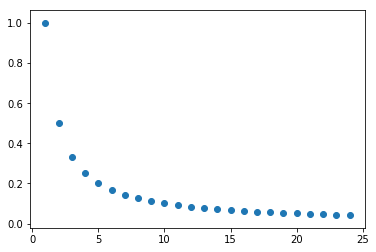We might want to combine this plot with another set of points. Let us create another set of data:

In :
zs = [1 / (25 - x) for x in xs]

In :
plt.scatter(xs, ys)
plt.scatter(xs, zs);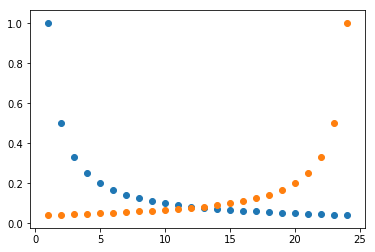We can add a legend to our plot (which can include LaTeX) as well as axes labels and a title:

In :
plt.scatter(xs, ys, label="$y=\\frac{1}{x}$")
plt.scatter(xs, zs, label="$y=\\frac{1}{25 - x}$")

plt.xlabel("$x$")
plt.ylabel("Value")
plt.title("My scatter plot")

plt.legend();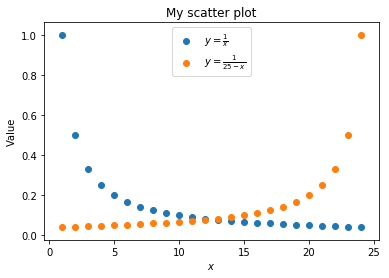Exercise

Plot a scatter plot with the following $x$, $y$ data:

In :
xs = range(200)
ys = [(100 - x) ** 2 for x in xs]


## Creating histograms¶

Let us create some random data sampled from the exponential distribution to use for a histogram:

In :
import random  # Allows us to create random data
number_of_data_points = 50000
data = [random.expovariate(lambd=.5) for _ in range(number_of_data_points)]


Let us know plot the histogram for this:

In :
plt.hist(data);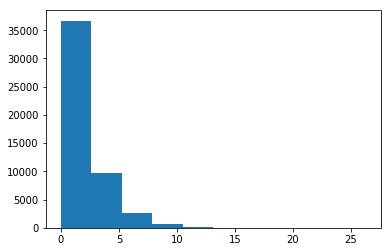We can change the number of bins and also specify that we would like the plot to be normalised (so as to show probabilities and not frequencies):

In :
plt.hist(data, bins=35, density=True);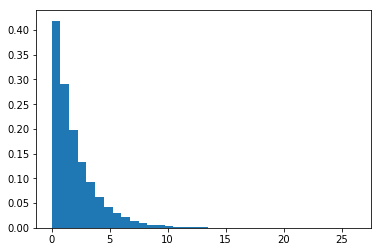It is known that the exponential distribution with rate $\lambda$ has probability distribution function (pdf):

$$f(x) = \lambda e ^{-\lambda x}$$

Let us include a line plot of that on our plot:

In :
import math

lambd = 0.5
values = range(16)
fs = [lambd * math.exp(- lambd * x ) for x in values]

plt.hist(data, bins=35, density=True)
plt.plot(values, fs);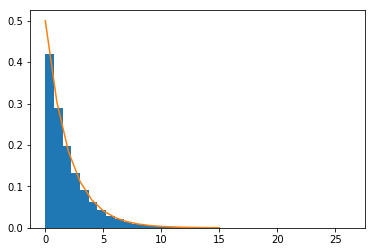Finally, we might want to save this figure and output it to a file:

In :
plt.hist(data, bins=35, density=True)
plt.plot(values, fs)
plt.savefig("the-exponential-distribution.pdf")By changing the file format name (.pdf, .png, .svg etc) we can change the format of the saved file.

EXERCISE Using the same code as for the scatter plots: add a title, axes labels and legend to the histogram.

EXERCISE Draw a histogram for randomly sampled data from the normal distribution (using random.normalvariate).

## Summary¶

In this section we have seen how to matplotib:

• To draw scatter plots;
• To draw histograms;
• To add labels and titles to plots;
• To save plots to a file.

This just touches on the capabilities of matplotlib.